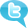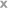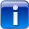NEWS: Chatbots.org survey on 3000 US and UK consumers shows it is time for chatbot integration in customer service!read more..

 Thompson Sampling: Adding positive rewards to Negative rewards in Python for Artificial Intelligence

 Posted: May 27, 2020

in Chapter 5 of AI Crash Course, the author writes

nSelected = nPosReward + nNegReward

for i in range(d):
print(‘Machine number ’ + str(i + 1) + ’ was selected ’ + str(nSelected) + ’ times’)
print(‘Conclusion: Best machine is machine number ’ + str(np.argmax(nSelected) + 1))

Why are the number of negative rewards added to the number of positive rewards? To find the best machine shouldn’t we only be concerned about the machine with the most positive rewards? I’m confused as to why we need to add the negative with the positive rewards. Also I understand that this is a simulation where you randomly assign successes and and you pre assign success rates. However in a real life situation, how do you know the success rates of each slot machine ahead of time? And how do you know which machines should be assigned a “1” ? Thank you so much! Here is the full code:

# Importing the libraries
import numpy as np

# Setting conversion rates and the number of samples
conversionRates = [0.15, 0.04, 0.13, 0.11, 0.05]
N = 10000
d = len(conversionRates)

# Creating the dataset
X = np.zeros((N, d))

for i in range(N):

for j in range(d):
if np.random.rand() < conversionRates[j]:
X[j] = 1

# Making arrays to count our losses and wins
nPosReward = np.zeros(d)
nNegReward = np.zeros(d)

# Taking our best slot machine through beta distribution and updating its losses and wins
for i in range(N):
selected = 0
maxRandom = 0

for j in range(d):
randomBeta = np.random.beta(nPosReward[j] + 1, nNegReward[j] + 1)
if randomBeta > maxRandom:
maxRandom = randomBeta
selected = j
if X[selected] == 1:
nPosReward[selected] += 1
else:
nNegReward[selected] += 1

# Showing which slot machine is considered the best

nSelected = nPosReward + nNegReward

for i in range(d):
print(‘Machine number ’ + str(i + 1) + ’ was selected ’ + str(nSelected) + ’ times’)
print(‘Conclusion: Best machine is machine number ’ + str(np.argmax(nSelected) + 1))